:

## Geometry of triangle

Maple

The geometry of the triangle
Romanova Elena,  8 class,  school 57, Kazan, Russia

Construction of triangle and calculation its angles

Construction of  bisectors

Construction of medians

Construction of altitudes

> restart:with(geometry):

The setting of the height of the triandle and let's call it "Т"
> triangle(T,[point(A,4,6),point(B,-3,-5),point(C,-4,8)]);

T

Construction of the triangle
> draw(T,axes=normal,view=[-8..8,-8..8]);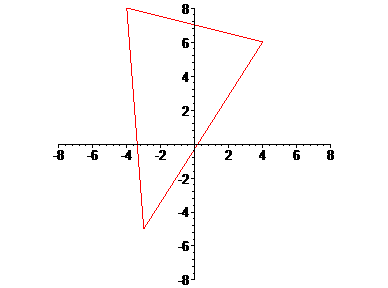Construction of the triangle АВС

> draw({T(color=gold,thickness=3)},printtext=true,axes=NONE);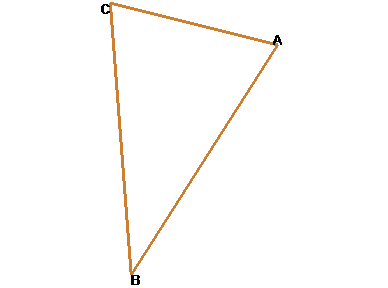Calculation of the distance between heights А and В - the length of a side АВ

> d1:=distance(A,B);

d1 := sqrt(170)

Calculation of the distance between heights В and С - the length of a side ВС
> d2:=distance(B,C);

d2 := sqrt(170)

The setting of line which passes through two points А and В
> line(l1,[A,B]);

l1

Display the equation of line l1
> Equation(l1);
> x;
> y;

-2 + 11 x - 7 y = 0

The setting of line which passes through two points А and С
> line(l2,[A,C]);

l2

Display the equation of line l2
> Equation(l2);
> x;
> y;

56 - 2 x - 8 y = 0

The setting of line which passes through two points В and С
> line(l3,[B,C]);

l3

Display the equation of line l3
> Equation(l3);
> x;
> y;

-44 - 13 x - y = 0

Check the point А lies on line l1
> IsOnLine(A,l1);

true

Check the point А lies on line l1
> IsOnLine(B,l1);

true

Calculation of the andle between lines l1 and l2
> FindAngle(l1,l2);

arctan(3)

The conversion of result to degrees
> b1:=convert(arctan(97/14),degrees);

97
arctan(--) degrees
14
b1 := 180 ------------------
Pi

Calculation of decimal value of this angle
> b2:=evalf(b1);

b2 := 81.78721981 degrees

Calculation of the andle between lines l1 and l3
> FindAngle(l1,l3);

arctan(3/4)

The conversion of result to degrees
> b3:=convert(arctan(97/99),degrees);

97
arctan(--) degrees
99
b3 := 180 ------------------
Pi

Calculation of decimal value of this angle
> b4:=evalf(b3);

b4 := 44.41536947 degrees

Calculation of the angle between lines l2 and l3
> FindAngle(l2,l3);

arctan(3)

The conversion of  result to degrees
> b5:=convert(arctan(97/71),degrees);

97
arctan(--) degrees
71
b5 := 180 ------------------
Pi

Calculation of decimal value of  this angle
> b6:=evalf(b5);

b6 := 53.79741070 degrees

Check the sum of all the angles of the triangle
> b2+b4+b6;

180.0000000 degrees

Analytical information about the point А
> detail(A);
name of the object: A
form of the object: point2d
coordinates of the point: [4, 6]
Analytical information about the point В
> detail(B);
name of the object: B
form of the object: point2d
coordinates of the point: [-3, -5]
Analytical information about the point С
> detail(C);
name of the object: C
form of the object: point2d
coordinates of the point: [-4, 8]

The setting of heights of the triangle points A,B,C and let's call it "Т"

with(geometry):
> triangle(ABC, [point(A,7,8), point(B,6,-7), point(C,-6,7)]):
The setting of the bisector of angle А in triandle АВС
> bisector(bA, A, ABC);

bA

Analytical information about the bisector of angle А in the triandle
> detail(bA);
name of the object: bA
form of the object: line2d
assume that the name of the horizonal and vertical                    axis are _x and _y
equation of the line: (15*170^(1/2)+226^(1/2))*_x+(-13*226^(1/2)-170^(1/2))*_y+97*226^(1/2)-97*170^(1/2) = 0

Construction of the triangle
> draw(ABC,axes=normal,view=[-8..8,-8..8]);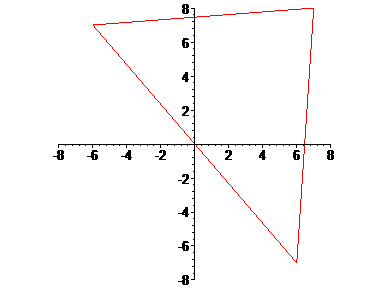Construction of the triangle ABC

> draw({ABC(color=gold,thickness=3)},printtext=true,axes=NONE);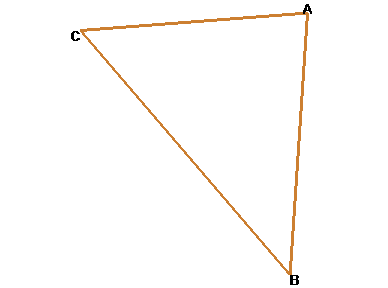Construction of the bisector of angle А

> draw({ABC(color=gold,thickness=3),bA(color=green,thickness=3)},printtext=true,axes=NONE);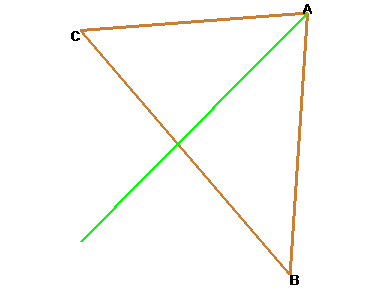The setting of the bisector of angle В in the triangle АВС

> bisector(bB, B, ABC);

bB

Analytical information about the bisector of angle B in the triandle
> detail(bB);
name of the object: bB
form of the object: line2d
assume that the name of the horizonal and vertical                    axis are _x and _y
equation of the line: (-15*340^(1/2)-14*226^(1/2))*_x+(-12*226^(1/2)+340^(1/2))*_y+97*340^(1/2) = 0

Construction of the bisector of angle В
>draw({ABC(color=gold,thickness=3),bA(color=green,thickness=3),bB(color=red,thickness=3)},printtext=true,axes=NONE);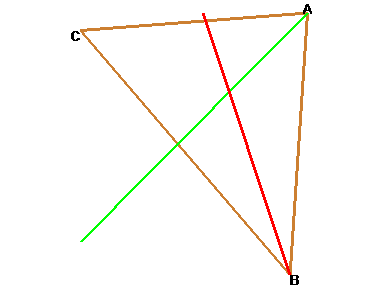The setting of the bisector of angle С in the triangle АВС

> bisector(bC, C, ABC);

bC

Analytical information about the bisector of angle С in the triangle
> detail(bC);
name of the object: bC
form of the object: line2d
assume that the name of the horizonal and vertical                    axis are _x and _y
equation of the line: (14*170^(1/2)-340^(1/2))*_x+(13*340^(1/2)+12*170^(1/2))*_y-97*340^(1/2) = 0

Construction of the bisector of angle С
>draw({ABC(color=gold,thickness=3),bA(color=green,thickness=3),bB(color=red,thickness=3),bC(color=blue,thickness=3)},printtext=true,axes=NONE);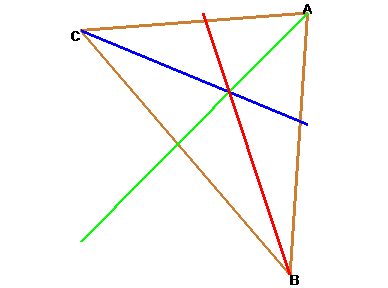Calculation of the point of intersection of the bisectors and let's call it "О"

> intersection(O,bA,bB,bC);coordinates(O);

O

7 sqrt(85) - 3 sqrt(2) sqrt(113) + 3 sqrt(85) sqrt(2)
[2 -----------------------------------------------------,
sqrt(85) sqrt(2) + sqrt(2) sqrt(113) + 2 sqrt(85)

-16 sqrt(85) - 7 sqrt(2) sqrt(113) + 7 sqrt(85) sqrt(2)
- -------------------------------------------------------]
sqrt(85) sqrt(2) + sqrt(2) sqrt(113) + 2 sqrt(85)

Construction of the bisectors and  marking of the point of intersection  "О" in the triandle
>draw({ABC(color=gold,thickness=3),bA(color=green,thickness=3),bB(color=red,thickness=3),bC(color=blue,thickness=3),O},printtext=true,axes=NONE);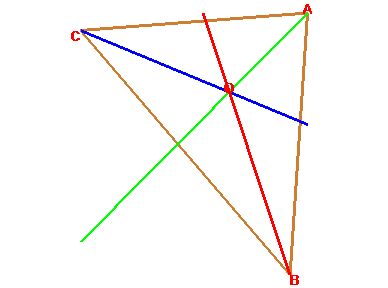> restart:
> with(geometry):
The setting of the heights of the triangle points A,B,C and let's call it "Т"
> point(A,7,8),point(B,6,-7),point(C,-6,7);

A, B, C

Let's call "Т1"
> triangle(T1,[A,B,C]);

T1

Construction of "Т1"
> draw(T1(color=gold,thickness=3),axes=NONE,printtext=true);The setting of the median from the point В in the trianglemedian(mB,B,T1,B1);
> median(mb,B,T1);

mB

mb

Construction of the median from the point В
> draw({T1(color=gold,thickness=3),mB(color=green,thickness=3),mb},printtext=true,axes=NONE);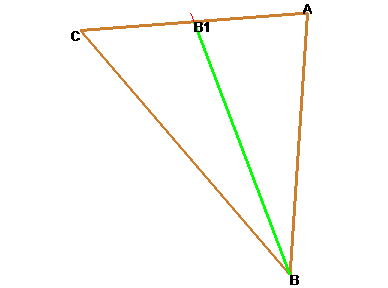The setting of the median from the point А in the trianglemedian(mA,A,T1,A1);
> median(ma,A,T1);

mA

ma

Construction of the median from the point А
>draw({T1(color=gold,thickness=3),mB(color=green,thickness=3),mA(color=magenta,thickness=3),ma},printtext=true,axes=NONE);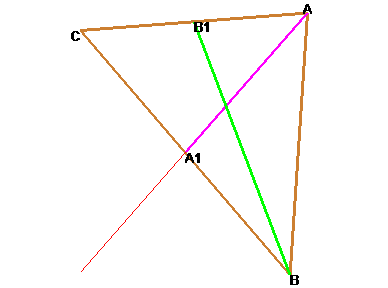The setting of the median from the point С in the trianglemedian(mC,C,T1,C1);
> median(mc,C,T1);

mC

mc

Costruction of the median from the point С
>draw({T1(color=gold,thickness=3),mB(color=green,thickness=3),mA(color=magenta,thickness=3),mA,mC(color=maroon,thickness=3)},printtext=true,axes=NONE);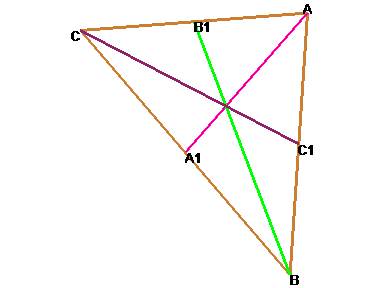Calculation of the point of  intersection of the median and let's call it "О"

>intersection(O,ma,mb,mC);coordinates(O);

O

[7/3, 8/3]

Construction of medians and marking of the point of  intersection "О" in the triangle
>draw({T1(color=gold,thickness=3),mB(color=green,thickness=3),mA(color=magenta,thickness=3),mA,mC(color=violet,thickness=3),O},printtext=true,axes=NONE);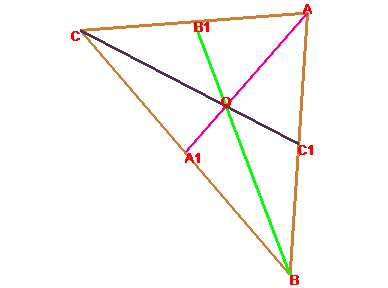> restart:with(geometry):
> _EnvHorizontalName:=x:_EnvVerticalName=y:       The setting of the heights of the triangle points A, B, C  and let's call it "Т"
> triangle(T,[point(A,7,8),point(B,6,-7),point(C,-6,7)]);

T

Construction of the triangle
> draw(T,axes=normal,view=[-8..8,-8..8]);The setting of the altitude in the triangle from the point Сaltitude(hC1,C,T,C1);
> altitude(hC,C,T);

hC1

hC

Analytical information about the altitude hC from the point С in the triangle
> detail(hC);
name of the object: hC
form of the object: line2d
assume that the name of the horizonal and vertical                    axis are _x and _y
equation of the line: -99+_x+15*_y = 0

Construction of the altitude from the point С
> draw({T(color=gold,thickness=3),hC1(color=green,thickness=3),hC},printtext=true,axes=NONE);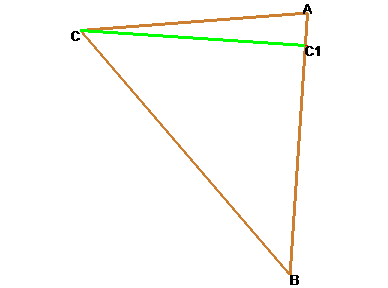The setting of the altitude in the triangle from the point Аaltitude(hA1,A,T,A1);
> altitude(hA,A,T);

hA1

hA

Analytical information about the altitude hA from the point А in the triangle
> detail(hA);
name of the object: hA
form of the object: line2d
assume that the name of the horizonal and vertical                    axis are _x and _y
equation of the line: -28-12*_x+14*_y = 0

Construction of the altitude from the point А
>draw({T(color=gold,thickness=3),hC1(color=green,thickness=3),hA1(color=red,thickness=3),hA1},printtext=true,axes=NONE);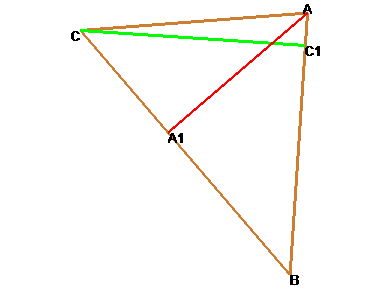The setting of the altitude from the point В

> altitude(hB1,B,T,B1);
> altitude(hB,B,T);

hB1

hB

Analytical information about the altitude hB from the point В in the triangle
> detail(hB);
name of the object: hB
form of the object: line2d
assume that the name of the horizonal and vertical                    axis are _x and _y
equation of the line: -71+13*_x+_y = 0

Consruction of the altitude from the point В
>draw({T(color=gold,thickness=3),hC1(color=green,thickness=3),hA1(color=red,thickness=3),hB1(color=blue,thickness=3),hB1},printtext=true,axes=NONE);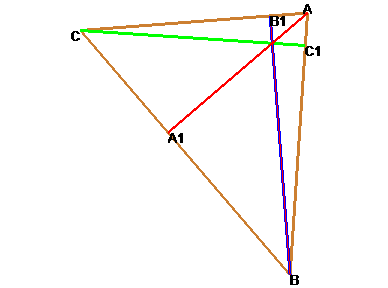Calculation of the point of intersection of altitudes and let's call it "О"

>intersection(O,hB,hA,hC);coordinates(O);

O

483  608
[---, ---]
97   97

Construction of altitudes and marking of the point of intersection "О" in the triangle
>draw({T(color=gold,thickness=3),hC1(color=green,thickness=3),hA1(color=red,thickness=3),hB1(color=blue,thickness=3),hB1,O},printtext=true,axes=NONE);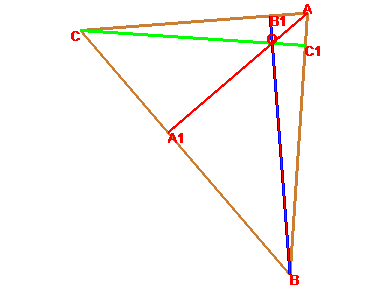﻿